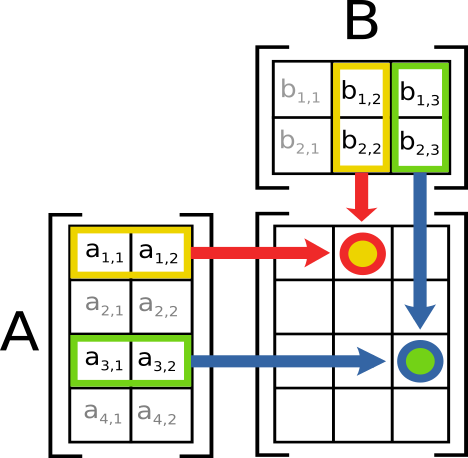# Matrix Multiplication For Dummies

Daniel Weibel
Created 16 Jul 2016

# How To Multiply Two Matrices?$m\times n$ matrix:

• $m$: number of rows (nrow)
• $n$: number of columns (ncol)

Multiplication of two matrices $m_1 \times n_1$ and $m_2 \times n_2$ is possible if either:

• $m_1 = n_2$ (i.e. nrow of 1st matrix = ncol of 2nd matrix)
• $n_1 = m_2$ (i.e. ncol of 1st matrix = nrow of 2nd matrix)

The resulting matrix always has:

• $m_1$ rows
• $n_2$ columns

# 1x4 and 4x2 Matrix

1x4
4x2 $\rightarrow$ OK

                        1  5    4x2 matrix
4  3
3  1
3  2
+------
1x4 matrix    4 2 5 2 |33 35    ==> Result: 1x2 matrix


# 4x1 and 4x2 Matrix

4x1
4x2 $\rightarrow$ Nope

                  1  5    4x2 matrix
4  3
3  1
3  2
+------
4x1 matrix    4 |
2 |
5 |
2 |         ==> Does not work!


# 1x4 and 2x4 Matrix

1x4
2x4 $\rightarrow$ Nope

                        1  4  3  3    2x4 matrix
5  3  1  2
+------------
1x4 matrix    4 2 5 2 |               ==> Does not work!


# 4x1 and 2x4 Matrix

4x1
2x4 $\rightarrow$ OK

                  1  4  3  3    2x4 matrix
5  3  1  2
+------------
4x1 matrix    4 |24 28 16 20
2 |12 14  8 10
5 |30 35 20 25
2 |12 14  8 10    ==> Result: 4x4 matrix


\hfil \textit{Swap first and second matrix of above multiplications.} \hfil

# 4x2 and 1x4 Matrix

4x2
1x4 $\rightarrow$ OK

                     4  2  5  2    1x4 matrix
+------------
4x2 matrix    1  5 |24 12 30 12
4  3 |28 14 35 14
3  1 |16  8 20  8
3  2 |20 10 25 10    ==> Result 4x4 matrix


# 4x2 and 4x1 Matrix

4x2
4x1 $\rightarrow$ Nope

                     4    4x1 matrix
2
5
2
+---
4x2 matrix    1  5 |
4  3 |
3  1 |
3  2 |      ==> Does not work!


# 2x4 and 1x4 Matrix

2x4
1x4 $\rightarrow$ Nope

                           4  2  5  2    1x4 matrix
+------------
2x4 matrix    1  4  3  3 |
5  3  1  2 |               ==> Does not work!


# 2x4 and 4x1 Matrix

2x4
4x1 $\rightarrow$ OK

                           4    4x1 matrix
2
5
2
+---
2x4 matrix    1  4  3  3 |33
5  3  1  2 |35    ==> Result: 2x1 matrix